Electron. J. Differential Equations, Vol. 2016 (2016), No. 231, pp. 1-9.

### Positive solutions of multi-point boundary value problems Youyuan Yang, Qiru Wang

Abstract:
This article concerns the boundary value problem consisting of the nonlinear differential equation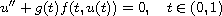and the multi-point boundary conditions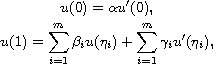where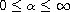,,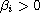,(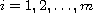). By using the fixed point index theory, we establish the existences of at least one positive solution and at least two positive solutions.

Submitted December 30, 2015. Published August 24, 2016.
Math Subject Classifications: 34B10, 34B18.
Key Words: Boundary value problems; multi-point boundary conditions; second-order nonlinear differential equations; positive solutions; fixed point index.

An addendum was posted on February 7, 2017. It points out some mistakes in this article; see the last page.

Show me the PDF file (216 KB), TEX file for this article.Youyuan Yang School of Mathematics Sun Yat-Sen University Guangzhou 510275, China email: yangyouyuan2016@163.com Qiru Wang School of Mathematics Sun Yat-Sen University Guangzhou 510275, China email: mcswqr@mail.sysu.edu.cn